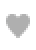# What is Confidence Interval?

Confidence interval is a metric to quantify the uncertainty in an estimated statistic (like mean of a given amount) when the real population parameter is unknown.

Confidence Interval is a range within which we are confident that the true value exists. The probability that the confidence interval contains the true parameter value is determined by the choice of a confidence level for the interval. The term "Confidence Interval" refers to the range of values used to extract specific, valuable information from population-based data with a high degree of confidence.

There are several institutes that provide the best machine learning course online. You can choose from them to learn more.

The term 'population parameter' is being used because, in most cases, you'll only have a small sample of the population to work with. The true population parameter (example: the average weight of all adult mice that exist) is rarely known, despite the fact that you can easily compute a sample statistic (example: the average weight of 10 adult mice) (not always). Confidence intervals, in simple terms, provide the upper and lower bounds of a given estimated statistic's range of possible values. The "margin of error" refers to the range of values within which a statistic can fall.

2 Types of Confidence Intervals Problems

There are mainly two types of problems where you would compute confidence intervals when you speak of confidence intervals. The formula for calculating the confidence interval varies depending on the type of study.

• The sample's 95% confidence interval.Using an example, calculate the confidence interval for the mean weight of mature white mice.
• Percentage of uncertainty. Using this example, calculate the confidence interval for the proportion of voters that cast their ballots for candidate A. (based only on exit polls data). Confidence intervals may be calculated using different formulas depending on the kind of issue.

Most of the time, you wouldn't know anything about the population's standard deviation since you'd only be dealing with a tiny sample. A T-distribution method might be appropriate in this situation. However, if you know the population standard deviation, you may use the Standard normal distribution-based technique instead.

If you don't understand what I'm saying, don't worry; I'll explain everything. A prior understanding of "Population parameter" and "Sample statistic," on the other hand, is required. A machine learning course online can help you to enhance your skills.

Difference Between Population Parameter vs Sample Statistic

A population parameter (such as the mean, standard deviation, and so on) is, as its name indicates, a value that may be derived from data collected from the complete population. A sample statistic, on the other hand, is based on a smaller subset of the whole population.

It is difficult to get or calculate the population parameter. If that were the case, we wouldn't spend so much time obsessing about numbers. Confidence intervals come into function here, after all. Since it is frequently not practicable to calculate the population parameter, we compute the statistic from a smaller sample and then estimate a confidence range within which the genuine population parameter may change.

A notable instance of this is during presidential / parliamentary elections. Here, the complete pool of voters in a country forms the population. And when the election is done, you may see exit polls results (flashing on TV/Internet) declaring a specified confidence interval percent for the win of a given candidate. These exit polls are in actuality done only on a smaller selection of voters. So, it is viewed as a sample statistic upon which the confidence intervals of likelihood of winning for a given candidate is assessed. By the way, elections are one of the rare situations when the population parameter itself is

truly measured.

You may have noticed that exit polls that are conducted in an objective manner often tend to accurately forecast the candidate who will end up winning. In certain circumstances, such as when there is a bias in the sampling procedure or when there is a significant overlap between the confidence ranges of candidates, there may be an exception to the rule. The best online data science courses can be helpful for you to get better insight on this topic.0Dailya RoyZupyak is a the world’s largest content marketing community, with over 300 000 members and 3 million articles. Explore and get your content discovered.